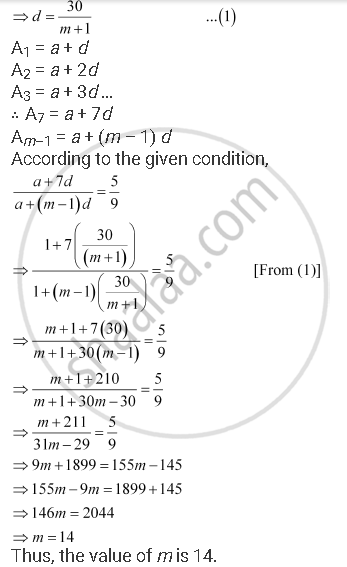CBSE (Arts) Class 11CBSE
Share

# Between 1 and 31, M Numbers Have Been Inserted in Such a Way that the Resulting Sequence is an A.P. and the Ratio of 7th and (M – 1)Th Numbers is 5:9. Find the Value of M. - CBSE (Arts) Class 11 - Mathematics

ConceptArithmetic Progression (A.P.)

#### Question

Between 1 and 31, m numbers have been inserted in such a way that the resulting sequence is an A.P. and the ratio of 7th and (m – 1)th numbers is 5:9. Find the value of m.

#### Solution

Let A1, A2, … Am be m numbers such that 1, A1, A2, … Am, 31 is an A.P.

Here, a = 1, b = 31, n = m + 2

∴ 31 = 1 + (m + 2 – 1) (d)

⇒ 30 = (m + 1) dIs there an error in this question or solution?

#### APPEARS IN

NCERT Solution for Mathematics Textbook for Class 11 (2018 to Current)
Chapter 9: Sequences and Series
Q: 16 | Page no. 185

#### Video TutorialsVIEW ALL 

Solution Between 1 and 31, M Numbers Have Been Inserted in Such a Way that the Resulting Sequence is an A.P. and the Ratio of 7th and (M – 1)Th Numbers is 5:9. Find the Value of M. Concept: Arithmetic Progression (A.P.).
S